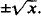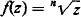# Multiple-Valued Function

(redirected from Multivalued function)
Also found in: Dictionary, Thesaurus, Wikipedia.
Related to Multivalued function: Single-valued function

## Multiple-Valued Function

a function that takes on several values for the same value of the argument. Multiple-valued functions arise when we invert single-valued functions whose values repeat. Thus, the function x2 takes on every positive value twice (for values of the argument differing only in sign); its inverse is the two-valued functionThe function sin x takes on each of its values an infinite number of times; its inverse is the infinite-valued function arcs in x. Multiple-valued functions play an important role in the theory of analytic functions of a complex variable. In the complex domain,has n values for any z ≠ 0, and f(z) = ln z, when z ≠ 0, has an infinite number of values.

Site: Follow: Share:
Open / Close